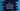# C++ to find the factorial of a number using class## C++ to find the factorial of a number using class:

In this post, we will learn how to find the factorial of a number using a class. Factorial of a number is equal to the multiplication of all numbers from 1 to that number.

Using a class, we can put all the factorial related operations in the class with a method to calculate the factorial. Then, we can create one object of the class and call the factorial function of that class to find the factorial.

Using a separate class is helpful to move all related functions in one place. With this program, you will learn how we can create a different class to calculate factorial.

### C++ program to calculate factorial using class:

``````#include <iostream>
using namespace std;

class Factorial
{
private:
int num;
int factorial = 1;

public:
void calculateFactorial();
void show();
};

void
Factorial::calculateFactorial()
{
cout << "Enter a number : " << endl;
cin >> num;

if (num == 0 || num == 1)
{
factorial = 1;
}
else
{
while (num > 1)
{
factorial = factorial * num;
num--;
}
}
}

void Factorial::show()
{
cout << "Factorial : " << factorial << endl;
}

int main()
{
Factorial factorial;
factorial.calculateFactorial();
factorial.show();
}``````

#### Explanation:

In this program:

• In this program, Factorial is a class to hold all factorial related functions.
• num and factorial are two int variables to hold the number and factorial value.
• calculateFactorial and show are two public functions. calculateFactorial is used to calculate factorial. calculateFactorial reads the user input number and calculates the factorial. It uses one while loop to calculate the factorial. It multiplies all numbers from num to 1.
• show is used to show the factorial value, i.e. factorial.

### Output:

``````Enter a number :
4
Factorial : 24

Enter a number :
5
Factorial : 120``````